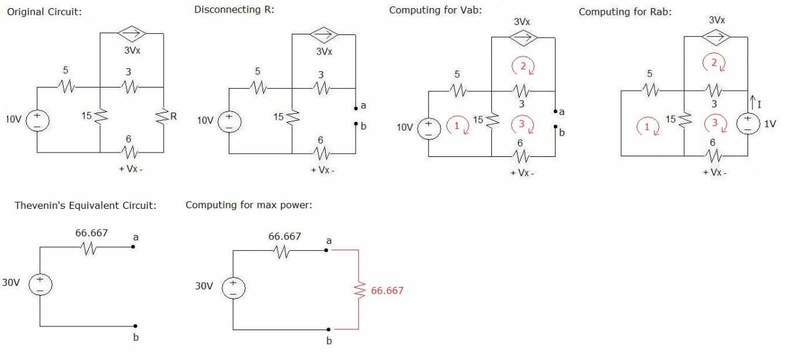# Maximum Power Transfer

## Homework Statement

Determine the value of RL that will draw the maximum power from
the rest of the circuit shown below. Calculate the maximum power. (This problem was included in our exam this week, and I don't really recall the exact values of the parameters, but I'm pretty sure that the circuit looks more or less like this.)V=IR
P=I2R
KVL

## The Attempt at a Solution

Computing for Vab:
mesh 1:
-10+5I1+15(I1-I3)=0
but I3=0, so I1=2A

mesh 2:
I2=3Vx
but Vx=-6I3, and I3=0, so I2=0

Since no voltage exists in the 3 and 6 ohm resistors, I shorted them and Vab will now be equal to the voltage across the 15 ohm resistor. (Will it? I'm not really sure about this)

Therefore,
V5ohm=Vab=15I1=15(2)
Vab=30V

Computing for Rab:

mesh 1:
20I1-153=0 --> eqn.1

mesh 2:
I2=3Vx
but Vx=-6I3
I2=3(-6I3)
I2+18I3=0 --> eqn.2

mesh 3:
-15I1-3I2+24I3=0 --> eqn. 3

I1=-0.011A
I2=0.270A
I3=-0.015A

I=-I3=-(-0.015)
I=0.015A

Rab=1/I=1/0.015
Rab=66.667ohms

Computing for the maximum power:

Pmax=I2Rab=(30/(66.667+66.667))2(66.667)
Pmax=3.375W

That is what I did. Sirs, did I solve it the right way? I'm especially uncertain about how I solved Vab.

#### Attachments

Last edited:

gneill
Mentor
The general method looks okay, but you've got a small problem with mesh1. The voltage is 10V and it's flowing through 5+15 = 20Ω. That should be a current of 0.5A, not 2A. As a result your Thevenin voltage does not turn out correctly.

Also, if I might make a suggestion that could simplify things a bit, why not first convert the 10V supply and its 5Ω series resistor to a Norton equivalent current? The Norton resistance will be in parallel with the 15Ω resistor so you can combine them. Then convert back to Thevenin. You'll have eliminated one mesh.

ah. thanks for pointing those out, sir. so it means that the major mistake is the 2A that's supposed to be 0.5A? nothing more?

gneill
Mentor
ah. thanks for pointing those out, sir. so it means that the major mistake is the 2A that's supposed to be 0.5A? nothing more?